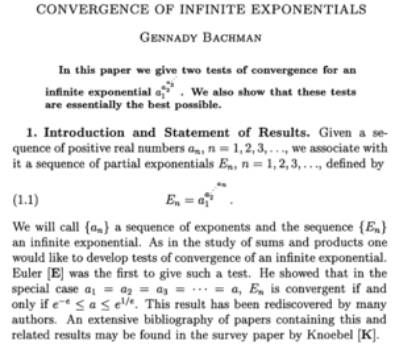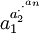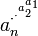A statement, that I made in October 2009 in Wikipedia , Talk:tetration

order of indexes in nested exponentials and infinite iterated exponentials

I'd like to repeat my criticism against the usual notation of indexes for the mentioned operations.

We find in the article:note the inverse indexing scheme

 Form Terminology$a^{a^{\cdot^{\cdot^{a^a}}}}$ Tetration$a^{a^{\cdot^{\cdot^{a^x}}}}$ Iterated exponentials$a_1^{a_2^{\cdot^{\cdot^{a_n}}}}$ Nested exponentials (also towers)$a_1^{a_2^{a_3^{\cdot^{\cdot^\cdot}}}}$ Infinite exponentials (also towers)

where it should be

 Form Terminology$a^{\cdot^{\cdot^{a^a}}}$ Tetration$a^{\cdot^{\cdot^{a^{a^x}}}}$ Iterated exponentials$a_n^{\cdot^{\cdot^{a_2^{a_1}}}}$ Nested exponentials (also towers)${\cdot^{\cdot^{a^{a^x}}}}$ infinitely iterated exponentials$\cdot^{\cdot^{\cdot^{a_3^{a_2^{a_1}}}}}$ Infinite exponentials (also towers)

The adaption of the order of indexes seems so natural to me, that I'm afraid I'm overlooking some special reason for that current use. For instance, the order of indexes which I claim to be wrong, can be found in Gennadi Bachman, "Convergence of infinite exponentials" (and in many more places. In the article of Ernst Schröder "Über iterirte Funktionen" (1871, Math Annalen) he begins his text with a recursive definition which suggests the non-inverted indexing scheme). However I cannot imagine one reason for this common (apparent inverse) indexing-scheme. So let me state my concern again.

We begin the operation at the top-level, so that should have index 1. ALternatively it should contain an initial value x from where the iterated operation starts (in the case of iterated exponentials, for tetration we assume x=1).

While this notational difference may be seen as neglectable for the finite case, this is not neglectable in the infinite case of iterated exponentiation. If the top-level is the dotted continuation, then there is no initial value x different from the iterated base a from where the operation could have started. If the top-level has x, and going to the bottom I assign an exponential base a to the previous value then I can eventually formally write the dots to the bottom, indicating, that I mean the infinite continuation of appending exponential bases.

Second: from this I'm tending to not to talk of "powertowers" here, but of "exponential-towers", since "power-towers" sounds more that I start with a value x, raise it to a power a, raise that to a power a and so on. While in tetration/iterated exponentiation I start with a value x, give it an exponential base a, give that an exponential base a and so on.

Schröder:Über iterirte Funktionen

Bachman:Convergence...

Gottfried Helms, Kassel